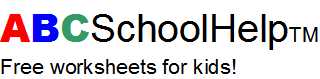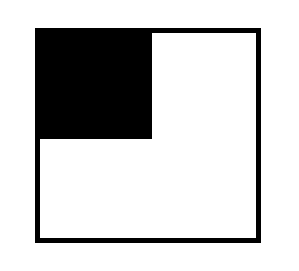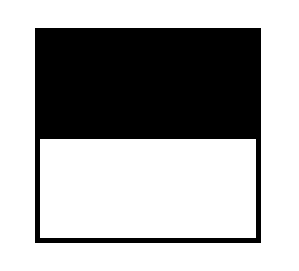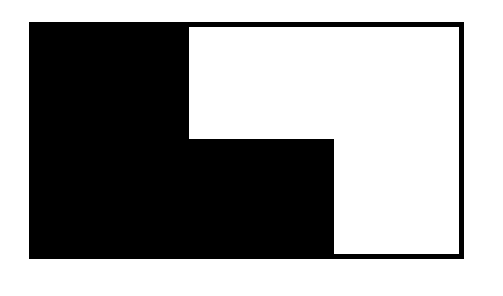Back | Print page | Home

# Grade 2 Math: Fractions and Decimals 2

```a. Write the fraction for the shaded area of this picture:b. Write the fraction for the shaded area of this picture:c. Write the fraction for the shaded area of this picture:d. How many of the following letters are X's?  Write a fraction to show this.

X X Y Y X Y    ____

e. How many of the following letters are X's?  Write a fraction to show this.

X Z Y Y X Y X Y Y Y X Y   ____

f. How many of the following letters are X's?  Write a fraction to show this.

Y Y Y Z Y    ____

```